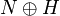# External semidirect product of Lie rings

## Definition

### Definition with the left action convention

Suppose$N$ is a Lie ring and$H$ is a Lie ring along with a homomorphism of Lie rings$\varphi$ from$H$ to the Lie ring of derivations$\operatorname{Der}(N)$. The external semidirect product of Lie rings, denoted$N \rtimes H$, is defined as follows:

• As an abelian group, it is the external direct product of$N$ and$H$, denoted$N \times H$ or$N \oplus H$.
• The Lie bracket is as follows:$[(n_1,h_1),(n_1,h_2)] = [n_1 + \varphi(h_1)n_2, h_1 + h_2]$

where$\varphi(h_1)n_2$ denotes the image of$n_2$ under the derivation$\varphi(h_1)$.

### Why the action convention does not matter

This is basically because every Lie ring is naturally isomorphic to its opposite Lie ring via the negative map.